1. /
2. CBSE
3. /
4. Class 06
5. /
6. Mathematics
7. /
8. NCERT Solutions for Class...

# NCERT Solutions for Class 6 Maths Exercise 5.4

NCERT solutions for Maths Understanding elementary## NCERT Solutions for Class 6 Maths Understanding elementary

###### Question 1.What is the measure of (i) a right angle? (ii) a straight angle?

(i) {tex}{90^ \circ }{/tex}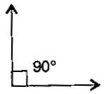(ii) {tex}{180^ \circ }{/tex}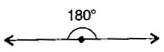NCERT Solutions for Class 6 Maths Exercise 5.4

###### Question 2.Say True or False:

(a)The measure of an acute angle <{tex}{90^ \circ }.{/tex}

(b)The measure of an obtuse angle <{tex}{90^ \circ }.{/tex}

(c)The measure of a reflex angle >{tex}{180^ \circ }.{/tex}

(d)The measure of on complete revolution= {tex}{360^ \circ }.{/tex}

(e)If {tex}m\angle {/tex}A = {tex}53^\circ {/tex} and {tex}m\angle {/tex}B = {tex}35^\circ ,{/tex} then {tex}m\angle {/tex}A >{tex}m\angle {/tex}B.

Answer: (a) True, (b) False, (c) True, (d) True, (e) True

###### Question 3.Write down the measure of:

(a) some acute angles (b) some obtuse angles

(give at least two examples of each)

(a) {tex}35^\circ ,20^\circ {/tex}

(b) {tex}110^\circ ,135^\circ {/tex}

###### Question 4.Measure the angles given below, using the protractor and write down the measure: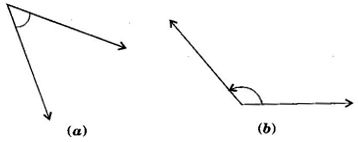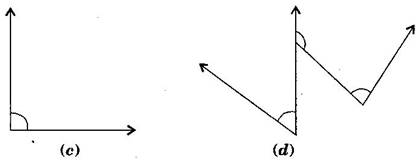(a) {tex}40^\circ {/tex}

(b) {tex}130^\circ {/tex}

(c) {tex}{90^ \circ }{/tex}

(d) {tex}{60^ \circ }{/tex}

NCERT Solutions for Class 6 Maths Exercise 5.4

###### Question 5.Which angle has a large measure? First estimate and then measure:

Measure of angle A =

Measure of angle B =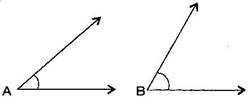Answer: {tex}\angle {/tex}B has larger measure.

{tex}\angle {/tex}A = {tex}40^\circ {/tex} and {tex}\angle {/tex}B = {tex}65^\circ {/tex}

###### Question 6.From these two angles which has larger measure? Estimate and then confirm by measuring them: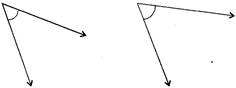Answer: Second angle has larger measure.

NCERT Solutions for Class 6 Maths Exercise 5.4

###### Question 7.Fill in the blanks with acute, obtuse, right or straight:

(a)An angle whose measure is less than that of a right angle is ________________.

(b)An angle whose measure is greater than that of a right angle is ________________.

(c)An angle whose measure is the sum of the measures of two right angles is ________________.

(d)When the sum of the measures of two angles is that of a right angle, then each one of them is ________________.

(e)When the sum of the measures of two angles is that of a straight angle and if one of them is acute then the other should be ________________.

Answer: (a) acute angle, (b) obtuse angle, (c) straight angle, (d) acute angle, (e) obtuse angle

###### Question 8.Find the measure of the angle shown in each figure. (First estimate with your eyes and then find the actual measure with a protractor).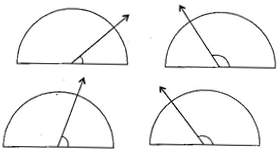(i){tex}{30^ \circ }{/tex}

(ii) {tex}{120^ \circ }{/tex}

(iii) {tex}{60^ \circ }{/tex}

(iv) {tex}{150^ \circ }{/tex}

NCERT Solutions for Class 6 Maths Exercise 5.4

###### Question 9.Find the angle measure between the hands of the clock in each figure: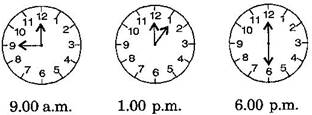(i){tex}{90^ \circ }{/tex} (Right angle)

(ii) {tex}{30^ \circ }{/tex} (Acute angle)

(iii) {tex}{180^ \circ }{/tex} (Straight angle)

###### Question 10.Investigate:

In the given figure, the angle measure {tex}{30^ \circ }.{/tex} Look at the same figure through a magnifying glass. Does the angle becomes larger? Does the size of the angle change?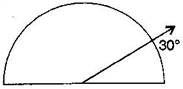Answer: No, the measure of angle will be same.

NCERT Solutions for Class 6 Maths Exercise 5.4

###### Question 11.Measure and classify each angle: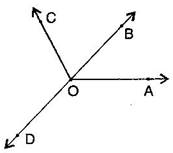Angle {tex}\angle {/tex}AOB {tex}\angle {/tex}AOC {tex}\angle {/tex}BOC {tex}\angle {/tex}DOC {tex}\angle {/tex}DOA {tex}\angle {/tex}DOB Measure Type

Sol.

 Angle {tex}\angle {/tex}AOB {tex}\angle {/tex}AOC {tex}\angle {/tex}BOC {tex}\angle {/tex}DOC {tex}\angle {/tex}DOA {tex}\angle {/tex}DOB Measure {tex}{40^ \circ }{/tex} {tex}{130^ \circ }{/tex} {tex}{90^ \circ }{/tex} {tex}{90^ \circ }{/tex} {tex}{140^ \circ }{/tex} {tex}{180^ \circ }{/tex} Type Acute Obtuse Right Right Obtuse straight

## NCERT Solutions for Class 6 Maths Exercise 5.4

NCERT Solutions Class 6 Maths PDF (Download) Free from myCBSEguide app and myCBSEguide website. Ncert solution class 6 Maths includes text book solutions from Class 6 Maths Book . NCERT Solutions for CBSE Class 6 Maths have total 14 chapters. 6 Maths NCERT Solutions in PDF for free Download on our website. Ncert Maths class 6 solutions PDF and Maths ncert class 6 PDF solutions with latest modifications and as per the latest CBSE syllabus are only available in myCBSEguide.

## CBSE app for Students

To download NCERT Solutions for Class 6 Maths, Social Science Computer Science, Home Science, Hindi English, Maths Science do check myCBSEguide app or website. myCBSEguide provides sample papers with solution, test papers for chapter-wise practice, NCERT solutions, NCERT Exemplar solutions, quick revision notes for ready reference, CBSE guess papers and CBSE important question papers. Sample Paper all are made available through the best app for CBSE students and myCBSEguide website.Test Generator

Create question paper PDF and online tests with your own name & logo in minutes.myCBSEguide

Question Bank, Mock Tests, Exam Papers, NCERT Solutions, Sample Papers, Notes

### 5 thoughts on “NCERT Solutions for Class 6 Maths Exercise 5.4”

1. Thanks

2. amazing

3. Thank for helping us
We really want like this apps.

4. Out standing ???

5. Very good